Electronic Circuit Simulation - Mesh Analysis [Python]
[Custom Thumbnail]

All the Code of the series can be found at the Github repository:
https://github.com/drifter1/circuitsim

Introduction

Hello it's a me again @drifter1! Today we continue with the Electric Circuit Simulation series, a tutorial series where we will be implementing a full-on electronic circuit simulator (like SPICE) studying the whole concept and mainly physics behind it! In this article we will cover the Mesh Analysis Method, which is based on Kirchhoff's Voltage Law (KVL) and is used to calculate the so called Mesh Currents.

Requirements:

• Physics and more specifically Electromagnetism Knowledge
• Knowing how to solve Linear Systems using Linear Algebra
• Some understanding of the Programming Language Python

Difficulty:

Talking about the series in general this series can be rated:
Today's topic(s) can be rated:
• Intermediate

Actual Tutorial Content

Mesh Analysis Method

Mesh (Current) Analysis is based on Kirchhoff's Voltage Law (KVL), which means that it's used to calculate the current in the various Loops of the Circuit. Thinking about the definition of Meshes, that we covered last time, Meshes are basically Loops without any other Loop inside of them. So, Mesh Analysis is a big improvement over KVL, as we will only have to find the so called mesh currents of each Mesh.

Mesh Current

So, what exactly is a mesh current? A mesh current (or loop current) is a current along each mesh/loop. This current flows clockwise or counterclockwise along the mesh/loop and this direction can be indicated by arrows. The following circuit diagram shows the mesh current along each mesh:[Image 1]

In this circuit there are two meshes and so we have two mesh currents: I1 and I2. Of course the current I1 flows through the elements Vs and R1. In the same way, current I2 flows through the circuit element R2. The current source on the right depends on the current value Ix, which makes it a dependent current source. But what is the value of Ix? (This is also the current that passes through R3) Well, this current of course has to flow in some direction! I1 flows downwards, whilst I2 flows upwards. Supposing that I1 > I2, we make Ix flow downwards. So, the value of this current is of course: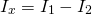It's worth mentioning that even if the hypothetical position is wrong, the result will still be right, but just negative! Seeing a negative current value we will know that the direction hypothesis was wrong and that the current actually flows in the opposite direction. As we can't know in which direction the current will flow in the end, we mostly tend to make all the currents flow in the same consistent direction, which can be clockwise or counterclockwise.

Mesh Analysis Steps

Remembering how KVL works (identify loops, assign currents, write KVL, solve system, solve for other currents and voltages using Ohm's Law) and tweaking it for Meshes only, the steps of Mesh Analysis are:
1. Identify the meshes of the circuit (loops with no other loops inside of them - sometimes called open windows of the circuit)
2. Assign current variables for each mesh (mesh currents), using some consistent direction (clockwise or counterclockwise)
3. Write the KVL equations for each mesh
4. Solve the resulting linear system for all mesh currents
5. Solve for other element currents and voltages you want using Ohm's Law

Mesh Analysis Example

Let's consider the following electronic circuit from Khan Academy: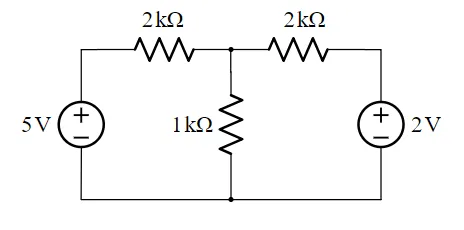[Image 2]

Let's apply Mesh Analysis to calculate the Current and Voltage of all Elements!

1. Identify Meshes

The Circuit has the following two meshes: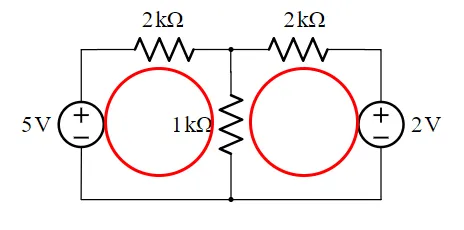2. Assign Mesh Currents

Let's assign mesh currents in the clockwise direction:Therefore, the current along each element is:So, we only have to calculate the two mesh currents: I1 and I2, as Ix can be derived from the equation:3. Write KVL Equations

Before we get into KVL, we should quickly recall the sign conventions for passive components:
• Going from "- → +" means rise or + in equation
• Going from "+ → -" means drop or - in equation
To get the voltage in the case of resistors we will have to use Ohm's law to write the voltage as the product of resistance times mesh current (V = R·I). The negative and positive poles of the circuit are: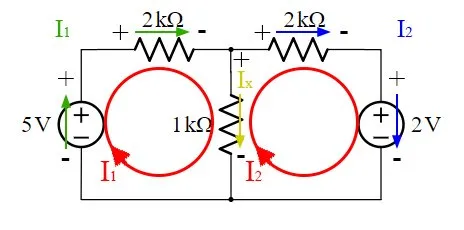In the same way for Mesh 2 we have:So, the final equation are: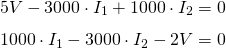4. Solve the Linear System

Let's solve the linear system now! Being a quite simple case, we can simply substitute one of the two variables into the other equation or just multiply the second one by 3 to cancel out I1 and calculate I2. Let's apply the second option:Adding these two equations we now get: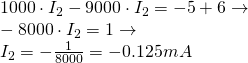Substituting the value of I2 into the first equation we can find I1:As you can see I2 came out negative and so the current flows in the opposite direction. Either way, we now have found the two most important currents, which are I1 and I2.

5. Solve for other Currents and Voltages

Substituting the two currents into the equation for Ix we can calculate this remaining current: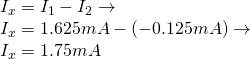Applying Ohm's Law for the Resistor in the middle, where we had the two mesh currents, we can calculate the voltage at the node that connects all three resistors, which is: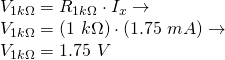Manual Python Implementation

To understand how we get into a Circuit Simulator, let's solve the previous problem using Python. Doing that you will understand why the method can't be automatized right now as it is! That's exactly why we will have to modify the methods a little bit...

Either way, the code that solves the problem looks like this:
import numpy as np
''' Electronic Circuit is ┌─ R1 ┬ R2 ─┐ V1 R3 V2 └─────┴─────┘ with following values: ''' V1 = 5 V2 = 2 R1 = 2000 # 2k Ohm R2 = 2000 # 2k Ohm R3 = 1000 # 1k Ohm
''' 1. Meshes Mesh 1: V1-R1-R3 Loop Mesh 2: R3-R2-V2 Loop
2. Mesh Currents (all clockwise) I1 for Mesh 1 I2 for Mesh 2 '''
# 3. Apply KVL for all Meshes # Mesh 1 # + V1 - R1·I1 - R3·(I1 - I2) = 0 # -(R1 + R3)·I1 + R3·I2 = - V1 I1_mesh1 = -(R1 + R3) I2_mesh1 = R3 b_mesh1 = -V1
# Mesh 2 # + R3·(I1 - I2) - R2·I2 - V2 = 0 # + R3·I1 - (R3 + R2)·I2 = V2 I1_mesh2 = R3 I2_mesh2 = -(R3 + R2) b_mesh2 = V2
# 4. Solve the Linear System # -3000 I1 + 1000 I2 = -5 # +1000 I1 - 3000 I2 = 2 a = np.array([[I1_mesh1, I2_mesh1],[I1_mesh2, I2_mesh2]]) b = np.array([b_mesh1, b_mesh2])
# Solve System x = np.linalg.solve(a,b) print(x)
# 5. Solve for other Currents and Voltages # The current that flows through R3 is Ix = x - x print("Ix = ", Ix) # The voltage along R3 is Vx = R3 * Ix print("Vx = ", Vx)

Running this code we get the same results as before: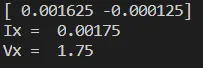To solve the system we used the numpy function solve() from linalg. To understand how we will get into an automation of the matrix/system creation later on, I also tried to show how the various coefficients of I1 and I2 are build up! You can see that the A-matrix contains only the Resistances, having the total resistance of each node in each cell, whilst the b-matrix contains only the Voltages. Using this stuff that we saw by Inspection we can modify the Mesh Analysis method. That's exactly what we will be doing next time we get into Mesh Analysis again!

RESOURCES

References:

3. https://www.electronics-tutorials.ws/dccircuits/dcp_5.html

Images:

1.Mathematical Equations were made using quicklatex

Final words | Next up on the project

And this is actually it for today's post! I hope that the Full-on example and "Manual" Python Implementation helped you understand what this method is all about!

Next up on this series is the solving method: Nodal Analysis.

So, see ya next time!

GitHub Account:

https://github.com/drifter1Keep on drifting! ;)

H2
H3
H4
3 columns
2 columns
1 column